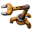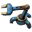## Add a slider to increase research and build times

Post your ideas and suggestions how to improve the game.
Kevin Ar18
InserterPosts: 20
Joined: Tue Jul 12, 2016 10:56 pm
Contact:

### Re: Add a slider to increase research and build times

tobsimon wrote:With different linear scalings for the different tiers, there will be pretty high steps between the tiers. I think a smooth scaling would be preferable.
Oh, yes, I know what you mean ... and it is not ideal to have it step like that.... If I could think of some solution without locking the settings to a particular scale (linear, super linear, exponential)....

tobsimon
Fast InserterPosts: 104
Joined: Wed Apr 29, 2015 10:58 am
Contact:

### Re: Add a slider to increase research and build times

Kevin Ar18 wrote:If I could think of some solution without locking the settings to a particular scale (linear, super linear, exponential)...
That's what I thought I suggested with the parametric exponent, though granted, my calculations were off.
tobsimon wrote:To make it simple for the user, I'd suggest two sliders for the factors at cost 50 (a) and 500 (b).
Then a function of the form f x^e with parameters f, e > 0 can be defined, which goes smoothly through
( 0 , 0 ) with slope 0 due to the function class and ( 50 , a*50 ), ( 500 , b*500 ) with a*50 < b*500, if
e = 1 + lg( b/a ) ,
f = a^2 /b /5^lg( b/a ) .
(lg is the base 10 logarithm, the formula for f was wrong before)

At cost 50, a is the factor roughtly at the transition from early to mid game and b at 500 from mid to late game.

With that, any linear scale is possible if a and b are the same, but also super and sub linear scales if they differ.
a=10, b=10 -> 10 x
a=100, b=100 -> 100 x
a=10, b=100 -> x^2 / 5.
a=100, b=10 -> illegal, b > a /10 needs to be fulfilled
a=6, b=2 -> rougthly 40 sqrt(x) (for an relatively easy late game: 100->400, 400->800, but 10->120 !!!)

Since the sliders are factors, they themself should employ a logarithmic scale, so that x100 is double the distance from x1 than x10. Alternitavely one can easily adjust the formular to work with absolute cost values instead of factors for the input. Even the reference costs 50 and 500 don't need to be pre-defined.

Side note:
Super linear is a pretty big class of functions and includes exponential. Apart from that, I think exponential is much too drastic, no matter how small the base, at some point it just goes through the roof. I think the desired groth rate is somewhere between x^1 and x^3, well within the realm of polynomial. As an alternative to function fitting, which might not behave well in all cases depending on the employed function class, there are many other methods for interpolation (e.g. spline interpolation), which stick closer to the corridor defined by the anchors, but have some problems beyond the set anchors.

### Who is online

Users browsing this forum: Impatient, narrowtux